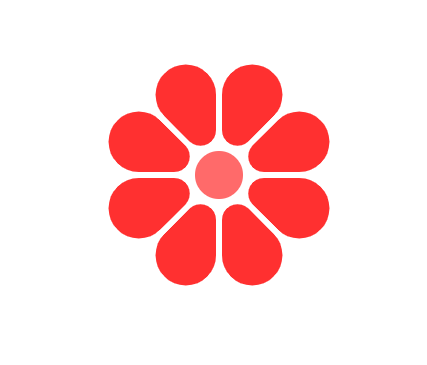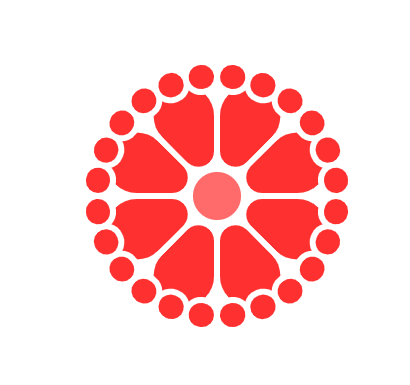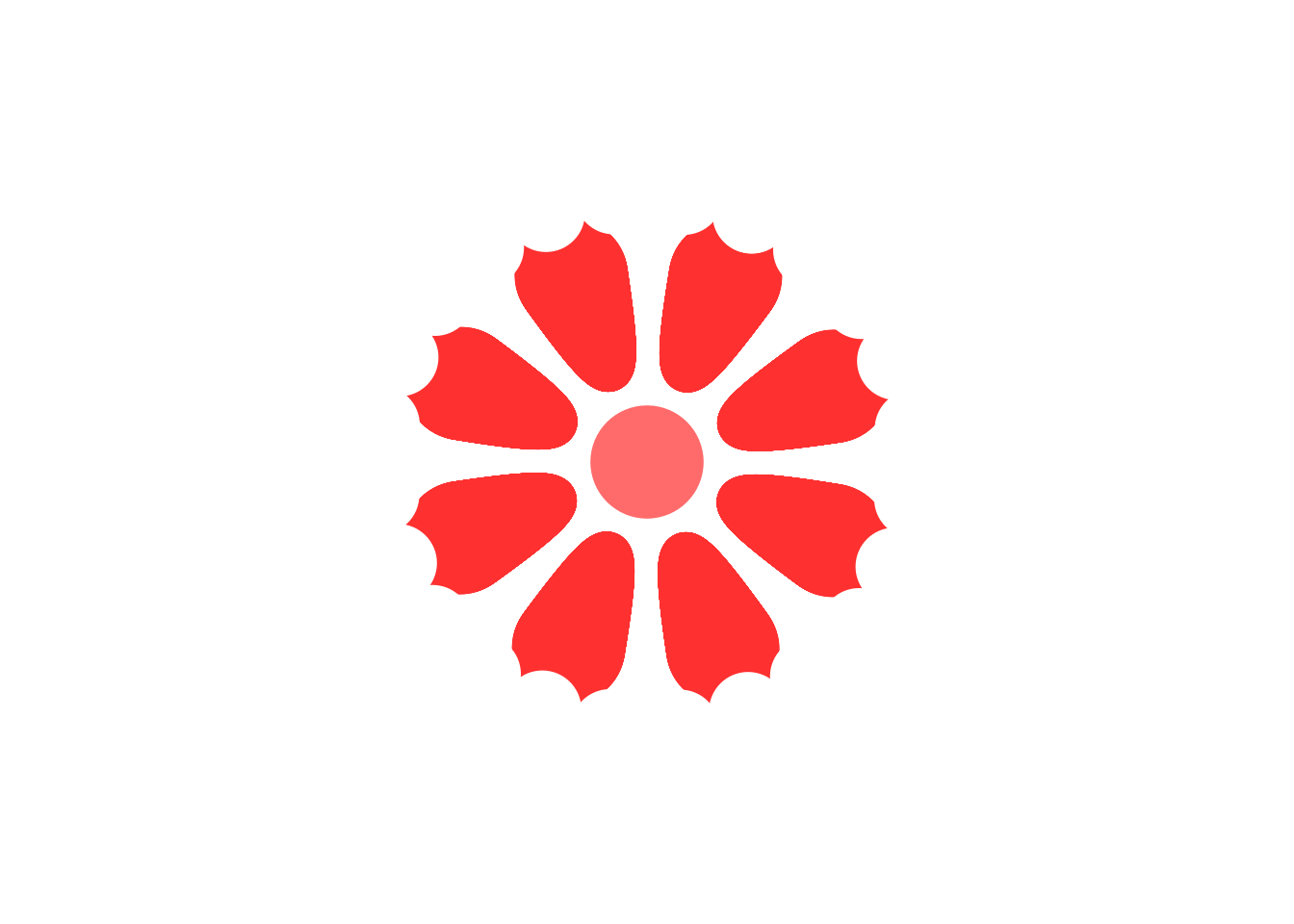## 思路#☝ 观察花的结构，由中心的圆点和八片花瓣组成。花瓣由内到外可视作圆圈从小到大一系列圆叠加而成，于是基本的思路是

• 用点图(`ggplot2::geom_point()`)作为绘图基本元素，并用使用极坐标`ggplot2::coord_polar()`绘制圆形坐标系。

• 分别构建花芯，花瓣两层所需数据，分别为df和df.middle，花芯为一个x=0,y=0一个点。花瓣坐标为x从1到8八瓣，y为从小到大的一个区间

• 反复尝试，调整花瓣的y取值范围，花芯大小 和`ggplot2::scale_size()`中点大小取值范围• 帖子最后还加了第三层外围的图层将花瓣外围修整为锯齿状，可分析为每片花瓣对应三个小点，于是创建了df.outer数据框记录第三层图层的点信息，并将其设为白色即可

• 最后使用 `theme_void()``theme(legend.position = "none")` 隐去坐标轴和图例。

## 最终代码和效果：#

``````# 加载ggplot2包
library(ggplot2)
# 生成花瓣坐标数据
# x为1到8，y为300到600的序列，步长为1，expand.grid生成x和y的所有组合
df=expand.grid(x=(1:8),y=seq(300,600,1))
# 点的大小为y平方，组成花瓣自内而外从小到大的圆
df\$size=df\$y^2
# 中间的点，坐标为0, 0
df.middle=data.frame(x=0,y=0,size=600^2)
# 外围的点，x为0到8，步长1/3的序列，y为800，每个花瓣对应三个点
df.outer=data.frame(x=seq(0,8,1/3),y=800,size=400^2)

ggplot(df, aes(x, y, size = size)) +  # ggplot，映射数据x,y,size至图上x,y,size三个属性
geom_point(color = "#FF3030") + # 花瓣
geom_point(data = df.middle, color = "#FF6A6A") + # 芯
geom_point(data = df.outer, color = "white") + # 外围的点为白色
lims(x=c(0,8),y=c(0,1100))+ # 设定坐标轴范围
scale_size(range = c(7, 20)) + # 设定点大小的范围
coord_polar(start = .4)+ # 设定极坐标和原点与12点钟方向的偏移量
theme_void()+theme(legend.position = "none") # 隐藏坐标轴和图例
``````